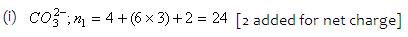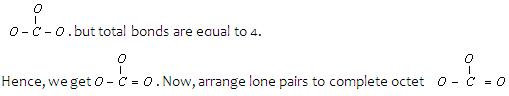1800-1023-196

+91-120-4616500

# Molecules Structures Construction And Poly Atomic Ions

## Molecules Structures Construction And Poly Atomic Ions

The following method is applicable to species in which the octet rule is not violated.

(i) Determine the total number of valence electrons in all the atoms present, including the net charge on the species (n1).

(ii) Determine n2 = [2 × (number of H atoms) + 8 × (number of other atoms)].

(iii) Determine the number of bonding electrons, n3, which equals n2 – n1. No. of bonds equals n3/2.

(iv) Determine the number of non-bonding electrons, n4, which equals n1 – n3. No. of lone pairs equals n4/2.

(v) Knowing the central atom (you’ll need to know some chemistry here, math will not help!), arrange and distribute other atoms and n3/2 bonds. Then complete octets using n4/2 lone pairs.

(vi) Determine the ‘formal charge’ on each atom.

(vii) Formal Charge = [valence electrons in atom) – (no. of bonds) – (no. of unshared electrons)]

(viii) Other aspects like resonance etc. can now be incorporated.

Illustrative examplesn2 = (2 × 0) + (8 × 4) = 32 (no. H atom, 4 other atoms (1’C’ and 3 ‘O’)

n3 = 32 – 24 = 8, hence 8/2 = 4 bonds

n4 = 24 – 8 = 16, hence 8 lone pairs.

Since carbon is the central atom, 3 oxygen atoms are to be arranged around it,

Thus,## NEET & AIIMS Exam Sample Papers

 AIIMS SAMPLE PAPERS View More NEET SAMPLE PAPERS View More